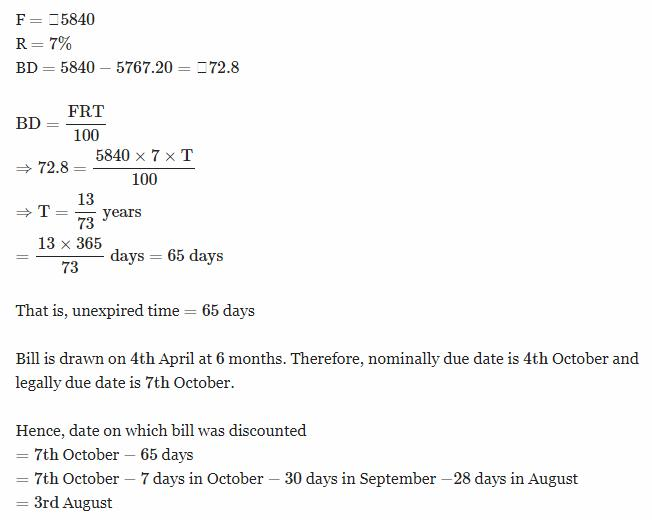Courses

# Test: Banker's Discount- 2

## 10 Questions MCQ Test UPSC CSAT: Logical Reasoning (LR) & Data Interpretation (DI) | Test: Banker's Discount- 2

Description
This mock test of Test: Banker's Discount- 2 for CAT helps you for every CAT entrance exam. This contains 10 Multiple Choice Questions for CAT Test: Banker's Discount- 2 (mcq) to study with solutions a complete question bank. The solved questions answers in this Test: Banker's Discount- 2 quiz give you a good mix of easy questions and tough questions. CAT students definitely take this Test: Banker's Discount- 2 exercise for a better result in the exam. You can find other Test: Banker's Discount- 2 extra questions, long questions & short questions for CAT on EduRev as well by searching above.
QUESTION: 1

### The banker's gain of a certain sum due 3 years hence at 10% per annum is Rs. 36. What is the present worth ?

Solution:

T = 3year
R = 10%
TD = (BG × 100)/TR
= (36 × 100)/(3 × 10)
= 12 × 10
= Rs.120
TD = (PW × TR)/100
⇒ 120 = (PW × 3 × 10)/100
⇒ 1200 = PW × 3
PW = 1200/3
= Rs.400

QUESTION: 2

### The present worth of a certain sum due sometime hence is Rs. 3400 and the true discount is Rs. 340. The banker's gain is:

Solution:

BG = (TD)2/PW
= (340)2/3400
= (340×340)/3400
= 340/10
= Rs.34

QUESTION: 3

### The banker's discount on Rs. 1600 at 15% per annum is the same as true discount on Rs. 1680 for the same time and at the same rate. What is the time?

Solution:

S.I. on Rs. 1600 = T.D. on Rs. 1680.
Rs. 1600 is the P.W. of Rs. 1680, i.e., Rs. 80 is on Rs. 1600 at 15%.
Time = (100*80)/(1600*15)
= 1/3 year
= 4 months.

QUESTION: 4

The banker's gain on a certain sum due 5/2 years hence is 9/25 of the banker's discount. What is the rate percent?

Solution:

Let BD = 1
Then, BG = 9/25
T = 5/2 years
TD = BD - BG
=> 1 - 9/25
=> 16/25
BG = (TD * RT)/100
9/25 = 16/25 * R * 5/2 * 1/100
R = 45/2
R = 22 1/2

QUESTION: 5

The banker's gain on a sum due 3 years hence at 12% per annum is Rs. 360. The banker's discount is:

Solution:

BG = Rs. 360
T = 3 years
R = 12%
TD = (BG×100)/TR
= (360×100)/(3×12)
= Rs. 1000
BG = BD - TD
⇒ BD = BG + TD
= 360 + 1000
= Rs. 1360

QUESTION: 6

The true discount on a certain sum due 6 months hence at 15% is Rs. 240. What is the banker's discount on the same sum for the same time at the same rate?

Solution:

Given in the question,
True Discount = 240
Rate of interest = 15%
Time = 6 months = 1/2 year
As we know the formula ,
True Discount = Banker's Gain ×    100
Time in years x Rate of Interest
⇒ 240 = Banker's Gain × 100/(1/2 × 15)
⇒ 240 = Banker's Gain × (100 x 2)/(1 × 15)
⇒ 24 = Banker's Gain × (10 x 2)/(1 × 15)
⇒ 24 = Banker's Gain × (2 x 2)/(1 × 3 )
⇒ 6 = Banker's Gain × 1/3
⇒ Banker's Gain = Rs. 6 x 3
⇒ Banker's Gain = Rs. 18
Apply the formula, As we know that ,
Banker's Gain = Banker's Discount − True Discount
⇒ 18 = Banker's Discount − 240
⇒ Banker's Discount = Rs. 258

QUESTION: 7

A bill is discounted at 10% per annum. If banker's discount is allowed, at what rate percent should the proceeds be invested so that nothing will be lost?

Solution:

Let the amount = Rs. 100
Then BD = Rs.10 (∵ banker's discount, BD is the simple Interest on the face value of the bill for unexpired time and bill is discounted at 10% per annum)
Proceeds = Rs. 100 - Rs. 10 = Rs. 90
Hence we should get Rs. 10 as the interest of Rs. 90 for 1 year so that nothing will be lost
⇒ 10 = (90×1×R)/100
⇒ R = (10×100)/90
= 100/9
= 11 1/9%

QUESTION: 8

The banker's discount on a sum of money for 3 years is Rs. 1116. The true discount on the same sum for 4 years is Rs. 1200. What is the rate percent?

Solution:

BD for 3 years = Rs.1116
BD for 4 years = (1116/3)×4
= Rs. 1488
TD for 4 years = Rs.1200
F = (BD×TD)/(BD−TD)
= (1488×1200)/(1488−1200)
= (1488×1200)/288
= (124×1200)/24
= (124×100)/2
= 62×100
= Rs.6200
⇒ Rs. 1488 is the simple interest on Rs. 6200 for 4 years
⇒1488 = (6200×4×R)/100
⇒ R = (1488×100)/(6200×4)
= (372×100)/(6200)
= 372/62
= 6%

QUESTION: 9

The true discount on a bill of Rs. 2160 is Rs. 360. What is the banker's discount?

Solution:

F = Rs. 2160
TD = Rs. 360
PW = F - TD = 2160 - 360 = Rs. 1800
True Discount is the Simple Interest on the present value for unexpired time
⇒ Simple Interest on Rs. 1800 for unexpired time = Rs. 360
Banker's Discount is the Simple Interest on the face value of the bill for unexpired time
= Simple Interest on Rs. 2160 for unexpired time
= (360/1800)×2160
= 1/5×2160
= Rs.432

QUESTION: 10

A banker paid Rs.5767.20 for a bill of Rs.5840, drawn of Apr 4 at 6 months. If the rate of interest was 7%, what was the day on which the bill was discounted?

Solution: